#### Determining yaw, pitch, and roll from a rotation matrix

It is often convenient to determine the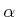,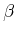, and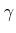parameters directly from a given rotation matrix. Suppose an arbitrary rotation matrix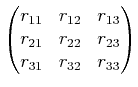(3.43)

is given. By setting each entry equal to its corresponding entry in (3.42), equations are obtained that must be solved for,, and. Note that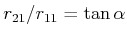and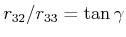. Also,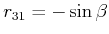and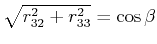. Solving for each angle yields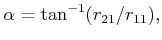(3.44)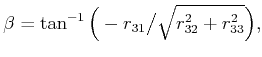(3.45)

and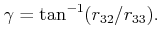(3.46)

There is a choice of four quadrants for the inverse tangent functions. How can the correct quadrant be determined? Each quadrant should be chosen by using the signs of the numerator and denominator of the argument. The numerator sign selects whether the direction will be above or below the-axis, and the denominator selects whether the direction will be to the left or right of the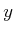-axis. This is the same as the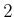function in the C programming language, which nicely expands the range of the arctangent to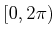. This can be applied to express (3.44), (3.45), and (3.46) as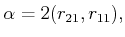(3.47)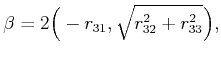(3.48)

and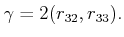(3.49)

Note that this method assumesand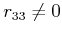.

Steven M LaValle 2020-08-14